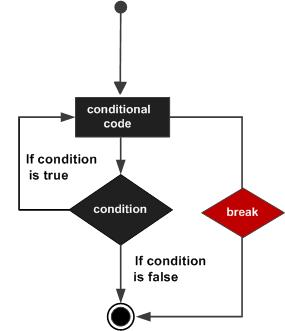# Break statement in java

The break statement in Java programming language has the following two usages −

• When the break statement is encountered inside a loop, the loop is immediately terminated and the program control resumes at the next statement following the loop.

• It can be used to terminate a case in the switch statement (covered in the next chapter).

## Syntax

The syntax of a break is a single statement inside any loop −

```break;
```

## Flow Diagram## Example

```public class Test {

public static void main(String args[]) {
int [] numbers = {10, 20, 30, 40, 50};

for(int x : numbers ) {
if( x == 30 ) {
break;
}
System.out.print( x );
System.out.print("\n");
}
}
}
```

This will produce the following result −

## Output

```10
20
```
java_loop_control.htm